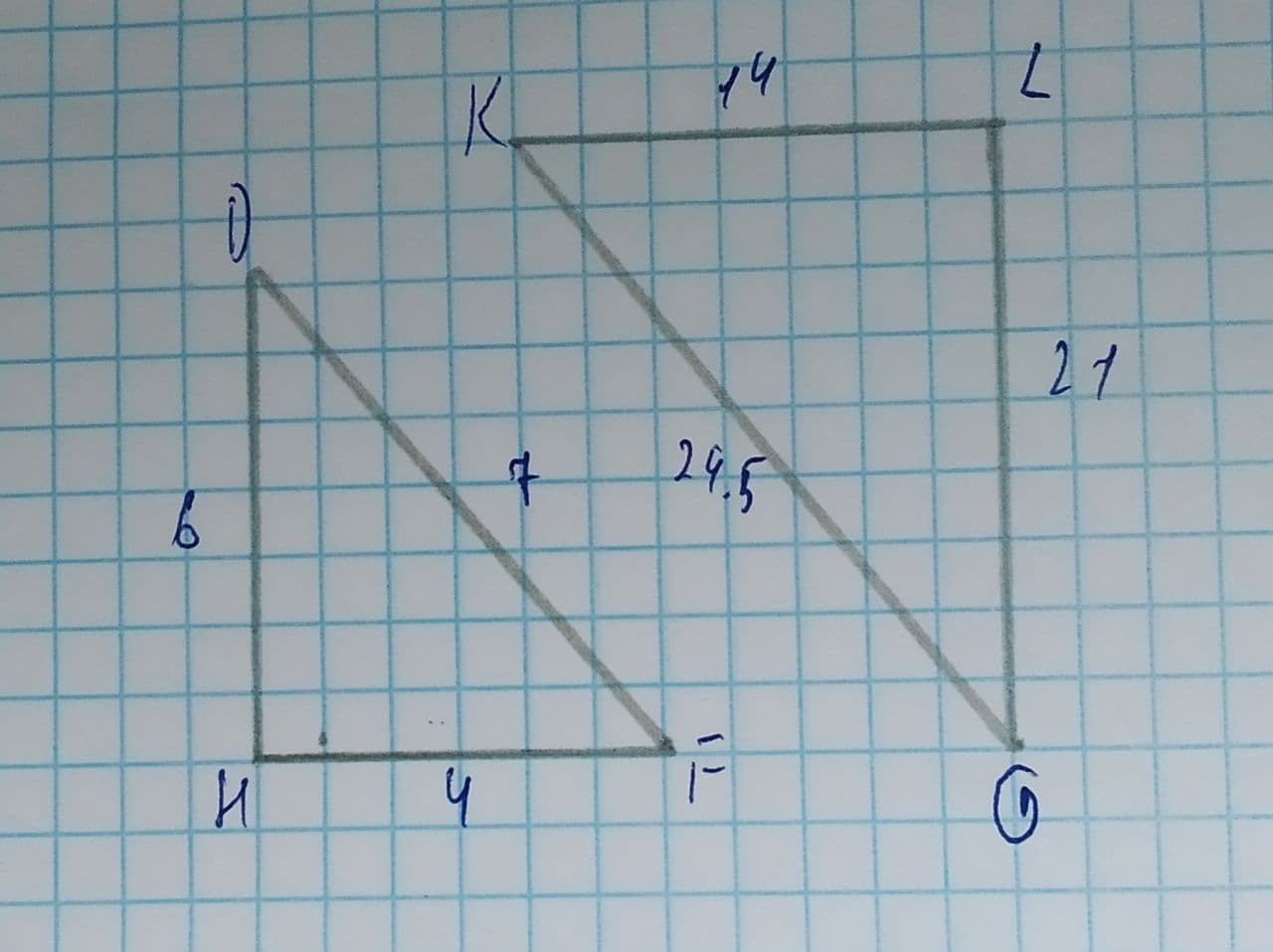Are the two triangles similar? If so, by what similarity shortcut?ddaeeric 2021-08-10 Answered
Are the two triangles similar? If so, by what similarity shortcut?SSS
SAS
AA
Not Similar

• Questions are typically answered in as fast as 30 minutes

Solve your problem for the price of one coffee

• Math expert for every subject
• Pay only if we can solve itlamusesamuset
Step 1:Concept
If sides of one triangle are proportional to sides of another triangle, triangles are similar by SSS.
Step 2:Solution
$$\displaystyle{\frac{{{K}{L}}}{{{H}{F}}}}={\frac{{{14}}}{{{4}}}}={3.5}$$
$$\displaystyle{\frac{{{K}{G}}}{{{D}{F}}}}={\frac{{{24.5}}}{{{7}}}}={3.5}$$
$$\displaystyle{\frac{{{G}{L}}}{{{D}{H}}}}={\frac{{{21}}}{{{6}}}}={3.5}$$
Triangles are similar by SSS